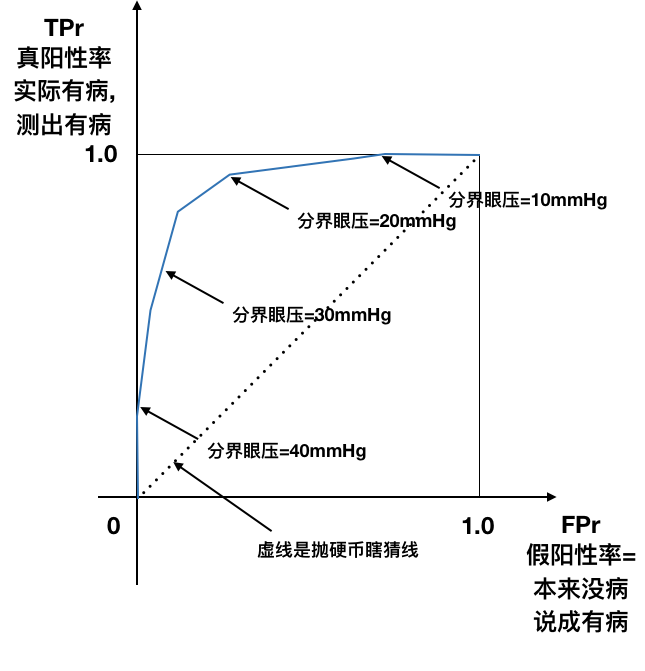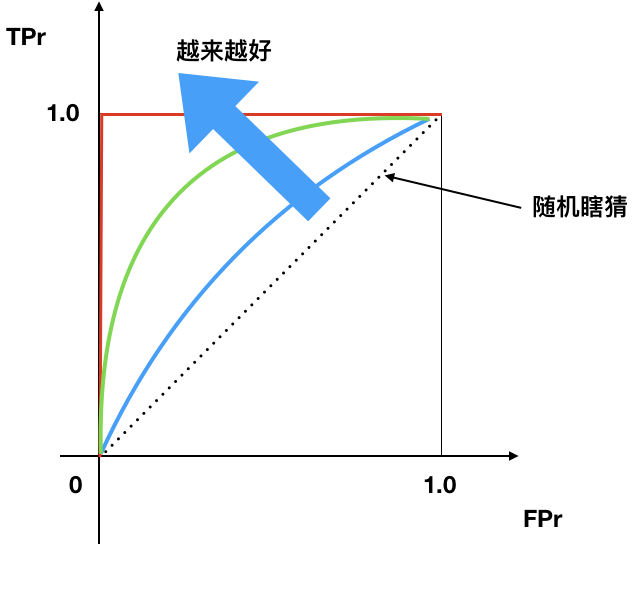# OpenCV C++学习笔记(3): 视频读写

VideoWriter::fourcc('m', 'p', '4', 'v')


# OpenCV C++学习笔记(2): Mat数据结构

opencv用了一种Mat的矩阵来存储图像数据, Mat看起来很神秘, 尤其是在处理图片局部的时候, 很神秘, 我一直想把两个摄像头拍摄的画面合并到一张图上显示, 查了很多, 才大概弄明白一点.

# OpenCV C++学习笔记(1): 读/写图像

《OpenCV 4计算机视觉项目实战》第二章, 代码在github

# AI 4 Med 笔记(1.2)各种率

### 各种率¶

• 真假阴阳各种率
• 敏感性和特异性
• 阳性预测率(PPV)和阴性预测率
• 置信区间, ROC曲线, F1 score

### Accuracy¶

• 正确率
• 有病没病都说对了的概率

$$Accuracy=\frac{100+110+120}{100+6+11+1+110+12+2+7+120}$$

### Recall¶

• 敏感性, 检出率, 真阳性率.
• 实际有病, 测出有病的概率

$$Recall=\frac{120}{2+7+120}$$

### Precision¶

• 精确度, 阳性预测率, PPV
• 测出有病, 还说对了的概率

$$Precision=\frac{120}{11+12+120}$$

### Specificity¶

• 特异性, 真阴性率
• 测出没病, 还说对了的概率

$$Specificity=\frac{100+6+1+110}{100+6+1+110+2+7}$$

### False Positive Rate¶

• 假阳性率, 误报率
• 本来没病, 测出有病的概率

$$False Positive Rate=\frac{11+12}{100+6+11+1+110+12}$$

### 真假阴阳性¶

• TP = true positive 真阳性
• FP = false positive (Type I error) 假阳性(Type I错误) , 印象里就是P<0.05的0.05
• TN = true negative 真阴性
• FN = false negative (Type II error)假阴性(Type II错误)

### 敏感特异性¶

• Accuracy: 分类正确的概率. 实际为(-)预测为(-), 实际为(+)预测为(+)叫做正确. $$Accuracy=\frac{TP + TN}{TP + TN + FP + FN}$$

• Classification error: (1-Accuracy), 分类错误的概率 $$Classification\; error=\frac{FP + FN}{TP + TN + FP + FN}$$

• Recall, 真阳性率: 实际上为(+), 能够被预测成(+)的概率

• Recall又叫
• True Positive Rate (TPR): 真阳性率
• Sensitivity: 敏感性
• Probability of detection: 检出率 $$Recall=\frac{TP}{TP+FN}$$
• Precision: 如果预测为(+), 那么预测正确的概率 $$Precision=\frac{TP}{TP+FP}$$

• False positive rate (FPR): 假阳性率, 误报率. 本来实际上是(-)的, 结果分类器报告成(+)的概率

$$False Positive Rate=\frac{FP}{TN+FP}$$
• Specificity, 特异性

### F1-score: 结合precision与recall¶

$$F_1=2\frac{precision \times recall}{precision+recall}=\frac{2TP}{2TP+FN+FP}$$

### F-score: 更一般地将precision与recall结合成单独一个数¶

$$F_\beta=(1+\beta^2)\frac{precision \times recall}{\beta^2\times precision+recall}=\frac{(1+\beta^2)TP}{(1+\beta^2)TP+FN+FP}$$

𝛽用来调整recall vs precision之间的重要程度:

• Precision-oriented users: 𝜷 = 0.5
• Recall-oriented users: 𝜷 = 2

### 阳性预测率 PPV¶

• Precision: 如果预测为(+), 那么预测正确的概率 $$Precision=\frac{TP}{TP+FP}$$
$$PPV = Precision = \frac{sensitivity \times prevalence}{sensitivity \times prevalence+(1 - specificity) \times (1 - prevalence)}$$

### ROC曲线¶

• X轴: False Positive Rate 假阳性率
• Y轴: True Positive Rate 真阳性率

• 理想点
• False positive rate 假阳性率=0
• True positive rate 真阳性率=1• ROC曲线越往左上, 越好.
• 曲线下面积(AUC), 越大越好
• 45度线是随机瞎猜线# AI 4 Med 笔记(1.1)胸片诊断

L(X,y)=

• -log(P(Y=1|X)) if y==1
• -log(P(Y=0|X)) if y==0